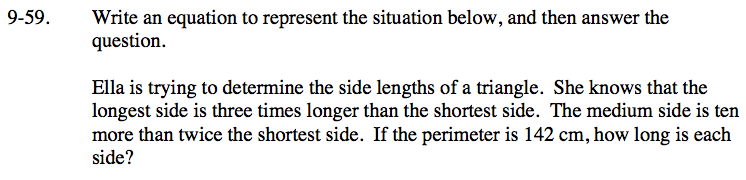### Home > CC3 > Chapter 9 > Lesson 9.2.1 > Problem9-59

9-59.
1. Write an equation to represent the situation below, and then answer the question.

2. Ella is trying to determine the side lengths of a triangle. She knows that the longest side is three times longer than the shortest side. The medium side is ten more than twice the shortest side. If the perimeter is 142 cm, how long is each side? Homework Help ✎Create a variable expression for perimeter to represent this problem with the shortest side defined by x.
How do the other sides relate to the shortest side, or x?

Shortest Side: x
Medium side: 10 + 2x
Longest Side: 3x
Add the lengths of the sides and set equal to the perimeter in order to solve for x.

Shortest Side: 22 cm
What are the lengths of the other sides?